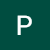# Hypothesis Testing

We often hear companies advertise dandruff shampoos that they claim are 100% effective, are these results realistically possible?

Hypothesis tests give a way of using samples to test whether statistical claims are likely to be true or not about a population.

A statistical measure for testing an assumption based on…

# Decision Trees

An introduction to Decision trees — a Machine Learning algorithm used as a foundation for complex ensemble techniques.

Decision Tree is a supervised learning algorithm used for predictive modeling and solving CART — classification and regression problems. It generates a tree in the form of simple ‘if-then’ rules where each…